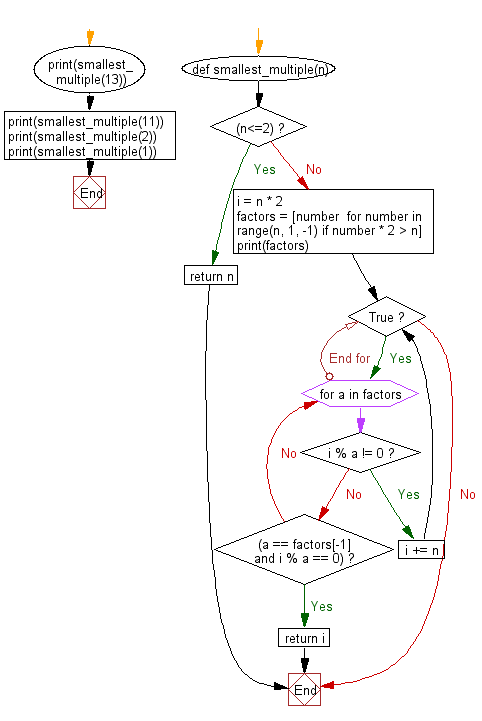﻿ Python Math: Find the smallest multiple of the first n numbers - w3resource# Python Math: Find the smallest multiple of the first n numbers

## Python Math: Exercise-10 with Solution

Write a Python program to find the smallest multiple of the first n numbers. Also, display the factors.

Sample Solution:-

Python Code:

``````def smallest_multiple(n):
if (n<=2):
return n
i = n * 2
factors = [number  for number in range(n, 1, -1) if number * 2 > n]
print(factors)

while True:
for a in factors:
if i % a != 0:
i += n
break
if (a == factors[-1] and i % a == 0):
return i

print(smallest_multiple(13))
print(smallest_multiple(11))
print(smallest_multiple(2))
print(smallest_multiple(1))
```
```

Sample Output:

```[13, 12, 11, 10, 9, 8, 7]
360360
[11, 10, 9, 8, 7, 6]
27720
2
1
```

Flowchart:## Visualize Python code execution:

The following tool visualize what the computer is doing step-by-step as it executes the said program:

Python Code Editor:

Have another way to solve this solution? Contribute your code (and comments) through Disqus.

What is the difficulty level of this exercise?

Test your Programming skills with w3resource's quiz.

﻿

## Python: Tips of the Day

Debug With the Print() Function:

```>>> for i in range(5):
...     print(i, end=', ' if i < 4 else '\n')
...
0, 1, 2, 3, 4
>>> for i in range(5):
...     print(f'{i} & {i*i}', end=', ' if i < 4 else '\n')
...
0 & 0, 1 & 1, 2 & 4, 3 & 9, 4 & 16
```